How to visualize interaction effectsI recently gave a workshop on data visualization. One of the topics was visualizing interaction effects (or “moderation” analyses). I think it is a great topic because it exemplifies quite well that there is not one solution to all problems. In general, I would argue that trying to visualize interaction effects is great idea. Due to the conditional nature of the effects obtained from standard regression analyses that include an interaction term, it is often hard to understand the direction and size of an interaction effect only based on regression results tables. In this post, I simply want to share some code that can be used to visualize a variety of different interaction effects.

Visualizing a 2×2 experimental design

``````# Loading packages
library(tidyverse)   # includes 'ggplot2' which is probably the best package for data viz
library(ggExtra)     # extends ggplot2

# Simulating the data
set.seed(100)
m1 <- sample(rep(c(0, 1), 250), 500) # Factor A
m2 <- sample(rep(c(0, 1), 250), 500) # Factor B
y <- 8 + -2*m1 + 2*m2 + 3*(m1*m2) + rnorm(500, 0, 2)
(data <- data.frame(m1 = factor(m1,
label = c("A1", "A2")),
m2 = factor(m2,
label = c("B1", "B2")),
y) %>%
as.tibble)
``````
```## # A tibble: 500 x 3
##    m1    m2        y
##    <fct> <fct> <dbl>
##  1 A2    B1     3.11
##  2 A1    B1     8.63
##  3 A2    B1     5.31
##  4 A1    B2     6.14
##  5 A1    B2    10.5
##  6 A2    B1     5.27
##  7 A2    B2    15.9
##  8 A1    B2    11.2
##  9 A1    B1     6.85
## 10 A2    B2    11.8
## # ... with 490 more rows
```

As we can see, we have a dataset that resembles data obtained from a 2×2 experimental design: `m1` and `m2` represent the experimental manipulations A and B resulting in four conditions (A1, A2, B1, B2). In such a case, were we are interesting in the effects of both conditions on the dependent variable `y` and in the effect of a potential interaction, a simple bar chart with a specific type of facetting is probably the best soluation.

``````ggplot(data,
aes(x = m1,
fill = m1,
y = y)) +
stat_summary(fun.y = mean,
geom = "bar") +
stat_summary(fun.data = mean_cl_normal,
geom="errorbar",
width = 0.25) +
ylim(0, 17) +
facet_wrap(~m2) +
labs(x = "Experimental Manipulations",
y = "Dependent Variable (M)") +
theme_minimal() +
scale_fill_brewer(palette = "Blues") +
theme(legend.position="none")
``````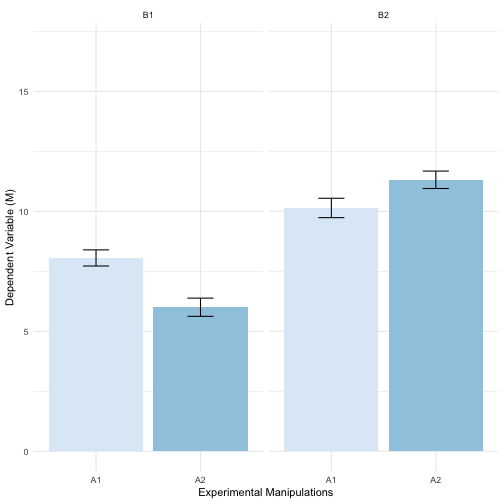An alternative is a modified line plot.

``````# Summarizing data
data2 <- data %>%
group_by(m1, m2) %>%
summarise(y_mean = mean(y),
y_se = psych::describe(y)\$se)

# Creating the plot
data2 %>%
ggplot(aes(x = m1,
y = y_mean,
color = m2)) +
geom_line(aes(group = m2)) +
geom_point() +
geom_errorbar(aes(ymin = y_mean-1.96*y_se,
ymax = y_mean+1.96*y_se),
width = .1) +
ylim(0, 17) +
labs(x = "Factor A",
color  = "Factor B",
y = "Dependent Variable") +
theme_minimal() +
scale_color_brewer(palette = "Blues")
``````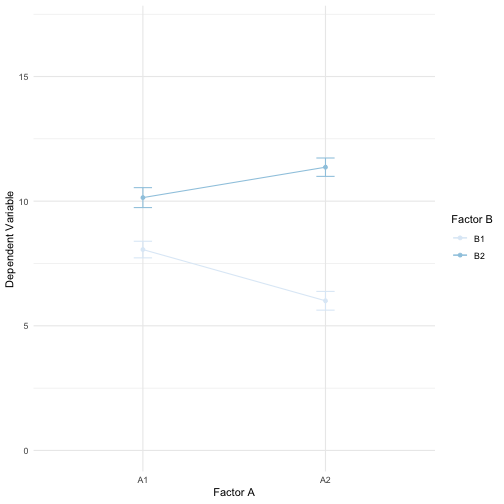Visualizing the moderation effect of a dichotomous variable

``````# Simulating the data
x <- rnorm(500, 2, 3)
m <- rep(c(0,1), 250)
y <- 5 + -4*x + 2*m + 4*(x*m) + rnorm(500, 0, 5)
(data <- data.frame(x, m = factor(m), y) %>%
as.tibble)
``````
```## # A tibble: 500 x 3
##        x m          y
##    <dbl> <fct>  <dbl>
##  1 5.29  0     -21.9
##  2 5.54  1       9.32
##  3 3.76  0      -6.41
##  4 5.23  1       9.05
##  5 5.41  0     -23.9
##  6 4.28  1       6.91
##  7 2.44  0       3.70
##  8 5.20  1       7.05
##  9 0.728 0       4.57
## 10 0.875 1       7.83
## # ... with 490 more rows
```

The moderator `m` here is a factor variable. The independent variable `x` and the dependent variable `y`, however, are continuous.

When the relationship of interested is between to continuous variables and only the moderator is dichotomous (e.g., gender, an experimental manipulation, intervention vs. no intervention,…), we can try to show as much information as possible and visualize the relationships fully using a colored scatterplot with separate regression lines for each group.

``````# Setting up the building blocks
basic_plot <- ggplot(data,
aes(x = x,
y = y,
color = m)) +
theme_bw() +
labs(x = "Independent variable",
y = "Dependent variable",
color = "Moderator")

# Colored scatterplot
basic_plot +
geom_point()
``````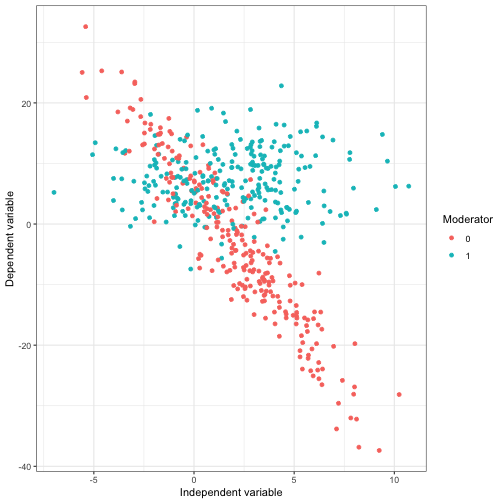``````# Colored scatterplot and regression lines
basic_plot +
geom_point(alpha = .3,
size = .9) +
geom_smooth(method = "lm")
``````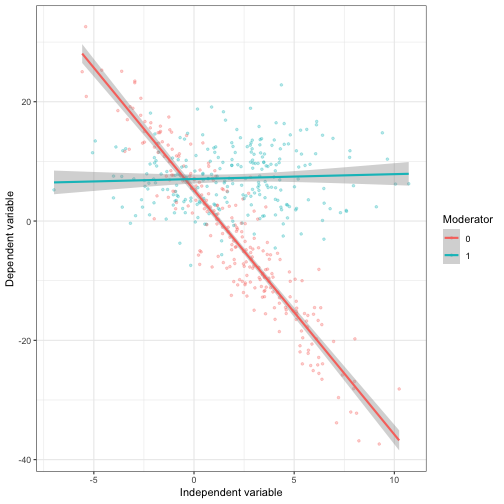``````ggsave("test2.png",
height = 4,
width = 5)

# Only regression lines
basic_plot +
geom_smooth(method = "lm",
se = F)
``````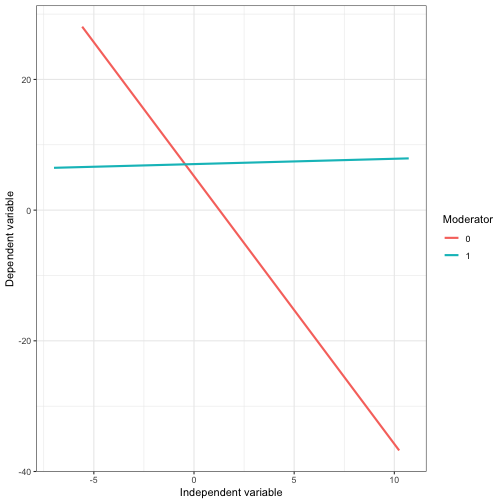Note: Many papers only show the last plot. However, I would always prefer the second plot which shows the actual scatterplots for each groups, the regression lines and confidence bounds.

Visualizing the moderation effect of metric variable

``````# Simulating the data
n <- 500
x <- runif(n)
m <- rnorm(n, 0.5, 1)
y <- 2*x + -2*m + -6*(x*m) + rnorm(500, 0, 5.88)
(data <- data.frame(x, m, y) %>%
as.tibble)
``````
```## # A tibble: 500 x 3
##         x       m       y
##     <dbl>   <dbl>   <dbl>
##  1 0.911   2.29   -19.5
##  2 0.352  -0.0184   3.78
##  3 0.409   0.754    3.61
##  4 0.867  -0.598    4.12
##  5 0.0416  0.629  -10.6
##  6 0.121   2.58   -10.0
##  7 0.265   1.43    -8.19
##  8 0.766   0.492   -0.599
##  9 0.405   0.410    3.19
## 10 0.290  -0.502    7.92
## # ... with 490 more rows
```

If the moderator (in this case `m`) is also continuous, visualizing interaction effects becomes more complex. There are generally three ways of presenting results from such a moderation analysis:

1. Estimating the regression for different values of the moderator (e.g., -SD, M, +SD) and reporting all three results in a table
2. Visualizing thee regression lines for three (arbitrary) groups, also know as the “pick-a-point” approach
3. Plotting the conditional effects for all values of the moderator (Conditional plot)

Pick-a-point approach

For this approach, we have to manually recode the moderator variable into three meaningful subgroups. Cutting points could be -SD, M, +SD or certain quantiles. In this case, we simply use the `cut` function to create subgroups1

``````data\$m_groups <- cut(data\$m, breaks = 3) %>%
factor(., labels = c("small", "average", "large"))
table(data\$m_groups)
``````
```##
##   small average   large
##      95     345      60
```

Next, we simply repeat the procedure that we already used for visualizing interaction effects with a dichotomuous moderator. We use the newly computed variable to color the points in the scatterplot and create separate regression lines.

``````ggplot(data,
aes(x = x,
y = y,
color = m_groups)) +
geom_point(size = .9,
alpha = .3) +
geom_smooth(method = "lm") +
theme_bw() +
scale_color_brewer(type = "qual",
palette = 3) +
labs(x = "Independent variable",
y = "Dependent variable",
color = "Moderator")
``````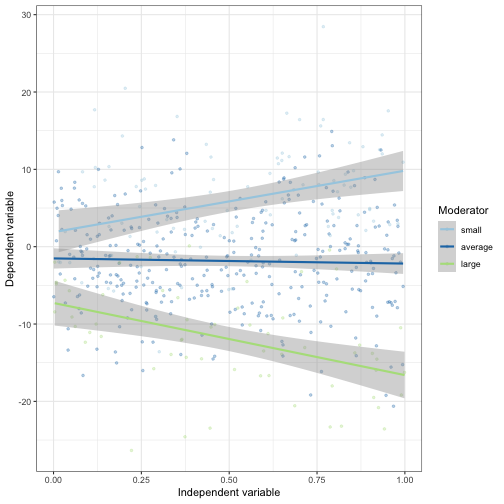Conditional effect plot

A problem with the pick-a-point approach is, however, that we only see the conditinal effects for three (somewhat arbitrary) groups. A conditional plot which visualizes the conditional effects for all possible values of the moderator is much more valuable.

For this approach, we first need to specify the interaction model itself.

``````model <- lm(y ~ x + m + x:m, data)
summary(model)
``````
```##
## Call:
## lm(formula = y ~ x + m + x:m, data = data)
##
## Residuals:
##      Min       1Q   Median       3Q      Max
## -18.8403  -3.8552  -0.2198   3.9432  17.5529
##
## Coefficients:
##             Estimate Std. Error t value Pr(>|t|)
## (Intercept)  -0.2601     0.5798  -0.449   0.6539
## x             2.3908     0.9955   2.402   0.0167 *
## m            -2.1500     0.4717  -4.558 6.51e-06 ***
## x:m          -6.0053     0.8369  -7.176 2.63e-12 ***
## ---
## Signif. codes:  0 '***' 0.001 '**' 0.01 '*' 0.05 '.' 0.1 ' ' 1
##
## Residual standard error: 5.869 on 496 degrees of freedom
## Multiple R-squared:  0.4768, Adjusted R-squared:  0.4736
## F-statistic: 150.6 on 3 and 496 DF,  p-value: < 2.2e-16
```

Now, we have to create a function that uses the output of this model and creates a data frame that includes the conditional effects for several values of the moderator.

``````# Function that estimates the conditinal effects for certain values of the moderator
conditional.effects <-  function(model, x, m, quantiles = 10){
interact = paste0(x,':',m)
beta.hat = coef(model)
covs = vcov(model)
z0  = quantile(model\$model[,m], seq(0 , 1, 1/quantiles))
dy.dx = beta.hat[x] + beta.hat[interact]*z0
se.dy.dx = sqrt(covs[x, x] + z0^2*covs[interact, interact] + 2*z0*covs[x, interact])
upr = dy.dx+1.96*se.dy.dx
lwr = dy.dx-1.96*se.dy.dx
data.frame(m = z0, b = dy.dx, lwr, upr)
}

(con_effects <- conditional.effects(model,
x = "x",
m = "m",
quantiles = 500) %>%
as.tibble)
``````
```## # A tibble: 501 x 4
##        m     b   lwr   upr
##  * <dbl> <dbl> <dbl> <dbl>
##  1 -2.54  17.6 12.3   22.9
##  2 -2.24  15.8 11.0   20.7
##  3 -2.20  15.6 10.8   20.4
##  4 -2.13  15.2 10.5   19.8
##  5 -2.10  15.0 10.4   19.6
##  6 -2.05  14.7 10.2   19.3
##  7 -1.85  13.5  9.23  17.7
##  8 -1.79  13.1  8.98  17.3
##  9 -1.70  12.6  8.56  16.6
## 10 -1.68  12.5  8.49  16.5
## # ... with 491 more rows
```

The dataframe includes the values of the moderator (`m`), the respective conditional effects (`b`) and their 95% confidence intervals. We can now use this data frame to create a new plot.

``````(mod_plot <- ggplot(con_effects,
aes(x = m,
y = b,
ymin = lwr,
ymax = upr)) +
geom_smooth(stat = "identity",
color = "black") +
geom_hline(yintercept = 0, linetype = "dashed", color = "darkgrey") +
theme_bw() +
labs(x = "Moderator",
y = "Conditional effect of x on y"))
``````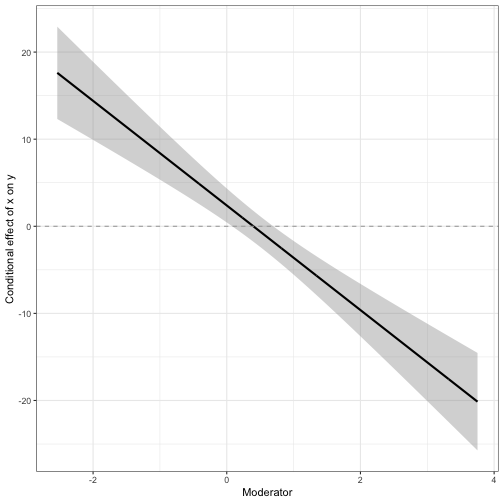This plot now shows the conditional effects for each value of the moderator. We can see that the effect of x on y is positive for lower values of `m` (the moderator) and turns negative for higher values of `m`. We can also asses at which values of `m`, the conditional effect of `x` on `y` becomes non-significant (Johnson-Neyman technique: see also https://rpubs.com/bachl/jn-plot).

I would suggest to also plot a histogram of the moderator so that one can assess how many values provide information for the information of the conditional effects.

``````ggMarginal(mod_plot,            # function of the package `ggExtra`
margins = c("x"),
type = "histogram",
color = "white",
fill = "darkgrey",
size = 3)
``````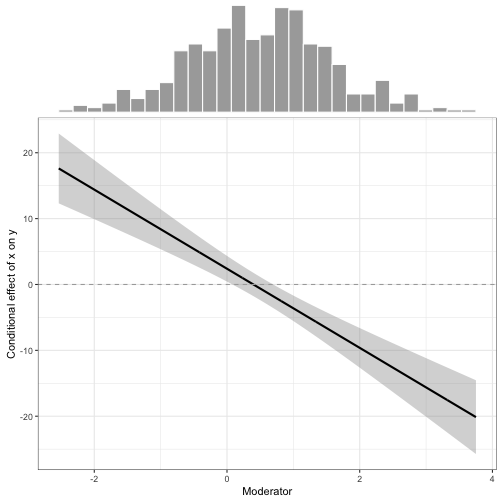Hope this is helpful to some people! 😉

1. For this function, we have to specify the breaks. When breaks is specified as a single number, the range of the data is divided into breaks pieces of equal length, and then the outer limits are moved away by 0.1% of the range to ensure that the extreme values both fall within the break intervals.